# cenkbircanoglu/similarityPyFetching latest commit…
Cannot retrieve the latest commit at this time.
Type Name Latest commit message Commit time
Failed to load latest commit information.imagesrequirementssettingssimilarityPytests.gitignore.travis.ymlLICENSEMANIFEST.inMakefileREADME.mdmake.batsetup.cfgsetup.pytox.ini

# Similarity Py## Installation

Install the package

`    \$ pip install similarityPy`

## Dependencies

``````enum
``````

###Distance Algorithms

#### Numerical Data

#####  Norm

#####  Manhattan Distance

Data: [{a, b, c}, {x, y, z}]
Formula: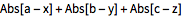#####  Euclidean Distance

Data: [{a, b, c}, {x, y, z}]
Formula: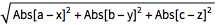#####  Squared Euclidean Distance

Data: [{a, b, c}, {x, y, z}]
Formula: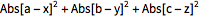#####  Normalized Squared Euclidean Distance

#####  Chessboard Distance

Data: [{a, b, c}, {x, y, z}]
Formula: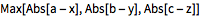#####  Bray Curtis Distance

Data: [{a, b, c}, {x, y, z}]
Formula: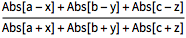#####  Canberra Distance

Data: [{a, b, c}, {x, y, z}]
Formula: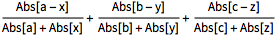#####  Cosine Distance

Data: [{a, b, c}, {x, y, z}]
Formula: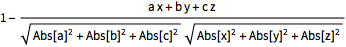#####  Correlation Distance

Data: [{a, b, c}, {x, y, z}]
Formula: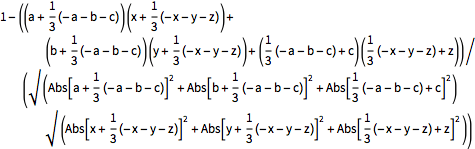#### Boolean Data

#####  Jaccard Dissimilarity

Data: [{True,False,True}, {True,True,False}]
Explanation:[u,v] is equivalent to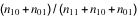, where nij is the number of corresponding pairs of elements in u and v respectively equal to i and j.

#####  Matching Dissimilarity

Data: [{True,False,True}, {True,True,False}]
Explanation:[u,v] is equivalent to (n10+n01)/Length[u], where nij is the number of corresponding pairs of elements in u and v respectively equal to i and j.

#####  Dice Dissimilarity

Data: [{True,False,True}, {True,True,False}]
Explanation:[u,v] is equivalent to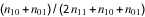, where nij is the number of corresponding pairs of elements in u and v respectively equal to i and j.

#####  Rogers Tanimoto Dissimilarity

Data: [{True,False,True}, {True,True,False}]
Explanation:[u,v] is equivalent to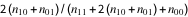, where nij is the number of corresponding pairs of elements in u and v respectively equal to i and j.

#####  Russell Rao Dissimilarity

Data: [{True,False,True}, {True,True,False}]
Explanation:[u,v] is equivalent to (n10+n01+n00)/Length[u], where nij is the number of corresponding pairs of elements in u and v respectively equal to i and j.

#####  Sokal Sneath Dissimilarity

Data: [{True,False,True}, {True,True,False}]
Explanation:[u,v] is equivalent to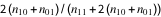, where nij is the number of corresponding pairs of elements in and respectively equal to i and j.

#####  Yule Dissimilarity

Data: [{True,False,True}, {True,True,False}]
Explanation:[u,v] is equivalent to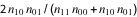, where nij is the number of corresponding pairs of elements in and respectively equal to i and j.

#### String Data

#####  Hamming Distance

Data: [{a, b, c}, {x, y, z}]
Explanation:[u,v] gives the number of elements whose values disagree in u and v.

#####  Edit Distance

Data: [{a, b, c}, {x, y, z}]
Explanation:[u,v] gives the number of one-element deletions, insertions, and substitutions required to transform u to v.

#####  Damerau Levenshtein Distance

Data: [{a, b, c}, {x, y, z}]
Explanation:[u,v] gives the number of one-element deletions, insertions, substitutions, and transpositions required to transform u to v.

#####  Needleman Wunsch Similarity (Not Implemented Yet)

Data: [{a, b, c}, {x, y, z}]
Explanation:[u,v] finds an optimal global alignment between the elements of u and v, and returns the number of one-element matches.

#####  Smith Waterman Similarity (Not Implemented Yet)

Data: [{a, b, c}, {x, y, z}]
Explanation:[u,v] finds an optimal local alignment between the elements of u and v, and returns the number of one-element matches.

##Testing

Run all tests:

`    \$ python -m unittest discover -s tests -p '*_test.py'`

Start test with nose and code coverage:

`    \$ nosetests --with-cov  --cov-report html  --cov  similarityPy tests/`
You can’t perform that action at this time.